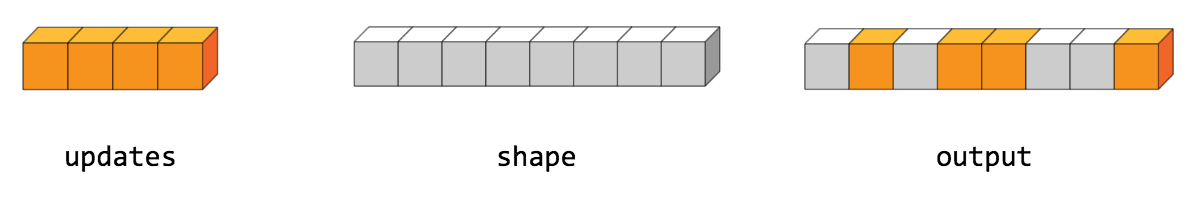Have a question? Connect with the community at the TensorFlow Forum

# tf.tensor_scatter_nd_update

Scatter `updates` into an existing tensor according to `indices`.

This operation creates a new tensor by applying sparse `updates` to the passed in `tensor`. This operation is very similar to `tf.scatter_nd`, except that the updates are scattered onto an existing tensor (as opposed to a zero-tensor). If the memory for the existing tensor cannot be re-used, a copy is made and updated.

If `indices` contains duplicates, then their updates are accumulated (summed).

`indices` is an integer tensor containing indices into a new tensor of shape `shape`. The last dimension of `indices` can be at most the rank of `shape`:

``````indices.shape[-1] <= shape.rank
``````

The last dimension of `indices` corresponds to indices into elements (if `indices.shape[-1] = shape.rank`) or slices (if `indices.shape[-1] < shape.rank`) along dimension `indices.shape[-1]` of `shape`. `updates` is a tensor with shape

``````indices.shape[:-1] + shape[indices.shape[-1]:]
``````

The simplest form of scatter is to insert individual elements in a tensor by index. For example, say we want to insert 4 scattered elements in a rank-1 tensor with 8 elements.In Python, this scatter operation would look like this:

``````    indices = tf.constant([, , , ])
updates = tf.constant([9, 10, 11, 12])
tensor = tf.ones(, dtype=tf.int32)
updated = tf.tensor_scatter_update(tensor, indices, updates)
with tf.Session() as sess:
print(sess.run(scatter))
``````

The resulting tensor would look like this:

``````[1, 11, 1, 10, 9, 1, 1, 12]
``````

We can also, insert entire slices of a higher rank tensor all at once. For example, if we wanted to insert two slices in the first dimension of a rank-3 tensor with two matrices of new values.

In Python, this scatter operation would look like this:

``````    indices = tf.constant([, ])
updates = tf.constant([[[5, 5, 5, 5], [6, 6, 6, 6],
[7, 7, 7, 7], [8, 8, 8, 8]],
[[5, 5, 5, 5], [6, 6, 6, 6],
[7, 7, 7, 7], [8, 8, 8, 8]]])
tensor = tf.ones([4, 4, 4])
updated = tf.tensor_scatter_update(tensor, indices, updates)
with tf.Session() as sess:
print(sess.run(scatter))
``````

The resulting tensor would look like this:

``````[[[5, 5, 5, 5], [6, 6, 6, 6], [7, 7, 7, 7], [8, 8, 8, 8]],
[[1, 1, 1, 1], [1, 1, 1, 1], [1, 1, 1, 1], [1, 1, 1, 1]],
[[5, 5, 5, 5], [6, 6, 6, 6], [7, 7, 7, 7], [8, 8, 8, 8]],
[[1, 1, 1, 1], [1, 1, 1, 1], [1, 1, 1, 1], [1, 1, 1, 1]]]
``````

Note that on CPU, if an out of bound index is found, an error is returned. On GPU, if an out of bound index is found, the index is ignored.

`tensor` A `Tensor`. Tensor to copy/update.
`indices` A `Tensor`. Must be one of the following types: `int32`, `int64`. Index tensor.
`updates` A `Tensor`. Must have the same type as `tensor`. Updates to scatter into output.
`name` A name for the operation (optional).

A `Tensor`. Has the same type as `tensor`.

[{ "type": "thumb-down", "id": "missingTheInformationINeed", "label":"Missing the information I need" },{ "type": "thumb-down", "id": "tooComplicatedTooManySteps", "label":"Too complicated / too many steps" },{ "type": "thumb-down", "id": "outOfDate", "label":"Out of date" },{ "type": "thumb-down", "id": "samplesCodeIssue", "label":"Samples / code issue" },{ "type": "thumb-down", "id": "otherDown", "label":"Other" }]
[{ "type": "thumb-up", "id": "easyToUnderstand", "label":"Easy to understand" },{ "type": "thumb-up", "id": "solvedMyProblem", "label":"Solved my problem" },{ "type": "thumb-up", "id": "otherUp", "label":"Other" }]